< img height="1" width="1" style="display:none" src="https://www.facebook.com/tr?id=282808403276418&ev=PageView&noscript=1" /> 挤压铝型材
+86-189 2311 6706（WhatsApp/Wechat)ISO9001认证挤压铝制造商

H汇信铝业有对菲律宾市场, 有着超过10年以上的丰富经验. 我们一直生产及出口菲律宾的热销系列, 例如 38, 50, 798, 900 等系列, 并支持各种流行的表面处理及颜色 powder coating, analok, hanalok 等, 为了满足不同客户的需求, 我们也提供定制服务.

### +铝型材

• #### 百叶窗框铝型材

##### 铝制百叶窗框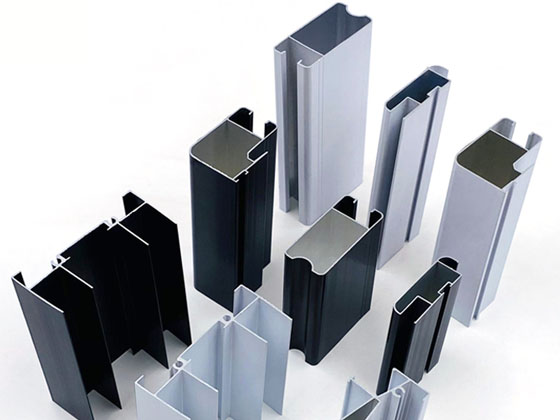• 原材料&热处理:铝合金& 6063-T5
• 表面处理:坯料，氧化，喷涂，电泳，木纹，抛光
• 颜色:
• Thicker Thickness:
• 涂层厚度:氧化:7~23μ, 喷涂:60~120μ, 电泳: 12~25μ
• 生产周期:大货20-25天，外加10天制作模具
• 起订量:每个型号500公斤
• 长度:5.8米， 或客户订制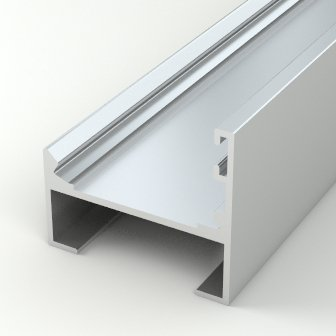FLB-065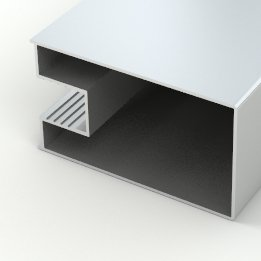FLB-001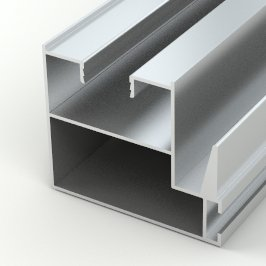FLB-002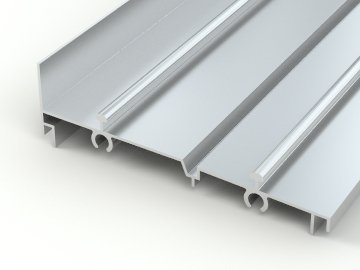FLB-003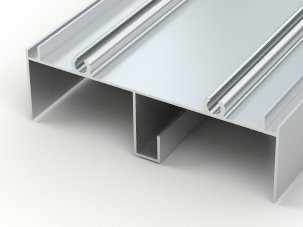FLB-004FLB-005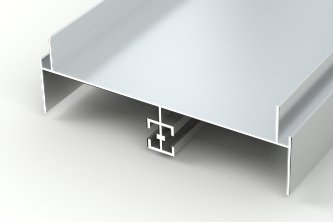FLB-006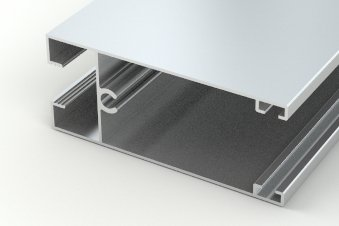FLB-007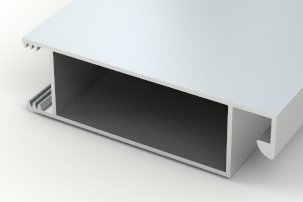FLB-008FLB-033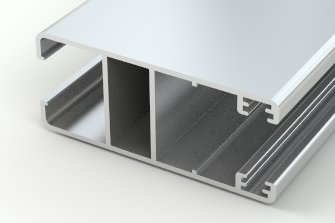FLB-060FLB-061FLB-062FLB-063FLB-065FLB-066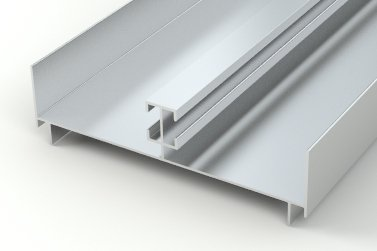FLB-069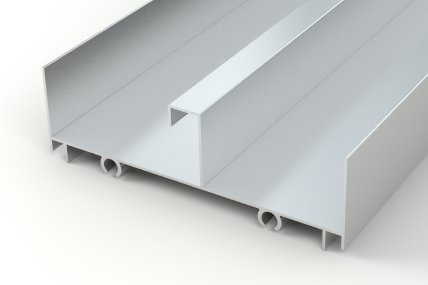FLB-070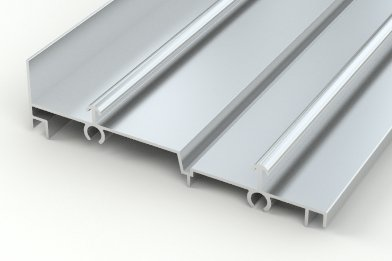FLB-071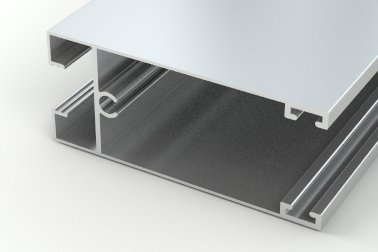FLB-074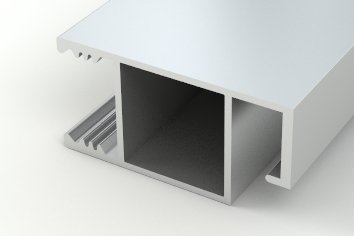FLB-075FLB-076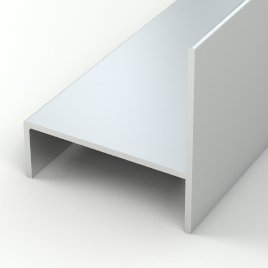FLB-077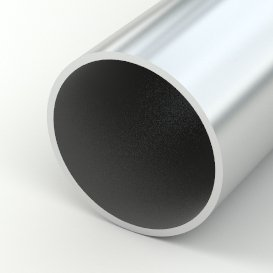FLB-078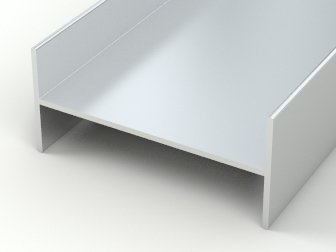FLB-082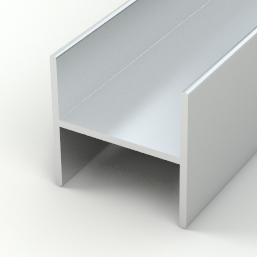FLB-083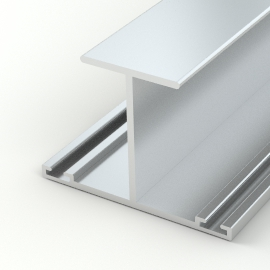FLB-085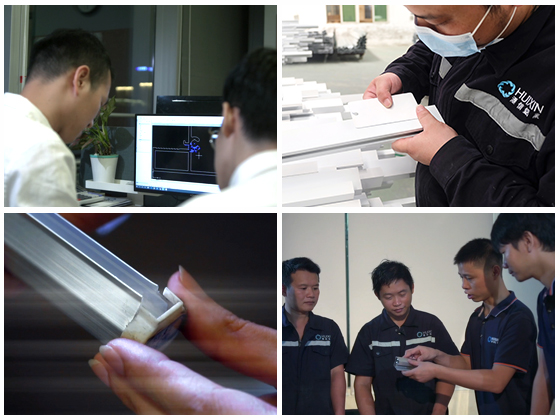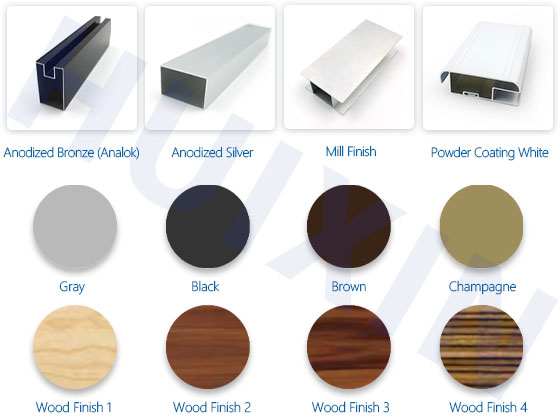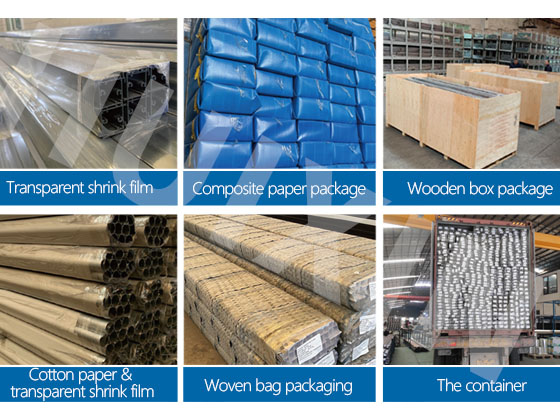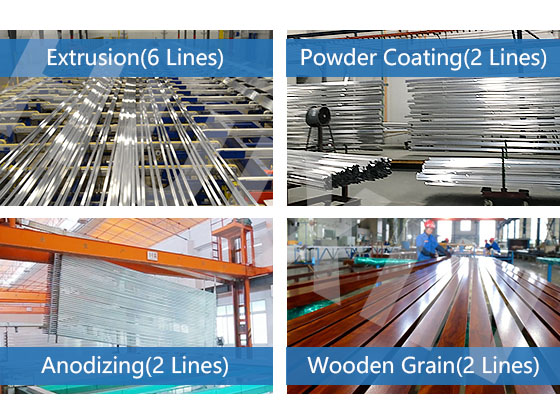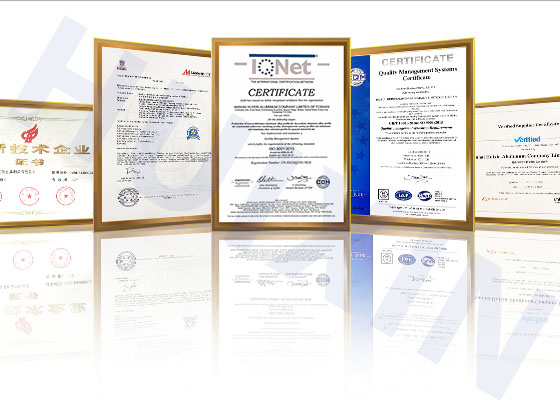ISO9001 认证公司

## 为什么汇信在全球范围内值得信赖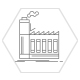10 年以上挤压铝生产经验 工厂专注于铝挤压行业的研发和制造10多年提供一站式 ODM & OEM 集成解决方案 从设计、生产、包装、检验和物流的优质服务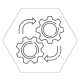3,000多套标准形状工装 拥有大量标准铝挤压产品模具，可为您节省模具成本内部模具制造及修整 内部模具制造及修整保证了模具尺寸的高精度并缩短了交货时间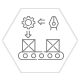先进的自动化生产设备 包括6条挤出生产线，2条粉末喷涂生产线，2条阳极氧化生产线，2条木纹生产线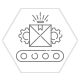年生产能力18000吨 强大的生产能力确保即使在旺季也能快速交货

## 完整生产线的一站式服务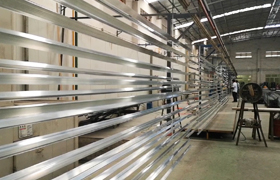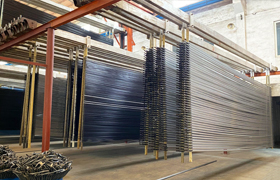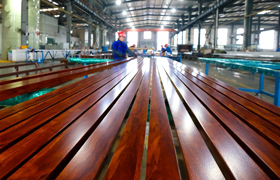挤出生产线 喷涂生产线 喷涂生产线 氧化生产线 木纹生产线

## 提交

• ---------
• 阿富汗
• 阿尔巴尼亚
• 澳大利亚
• 巴西
• 加拿大
• 中国
• 法国
• 德国
• 意大利
• 西班牙
• 瑞士
• 英国
• 美国
• ---------
• 阿富汗
• 阿尔巴尼亚
• 阿尔及利亚
• 美属萨摩亚
• 安道​​尔
• 安哥拉
• 安圭拉
• 安提瓜和巴布达
• 阿根廷
• 亚美尼亚
• 阿鲁巴岛
• 澳大利亚
• 奥地利
• 阿塞拜疆
• 巴哈马
• 巴林
• 孟加拉国
• 巴巴多斯
• 白俄罗斯
• 比利时
• 伯利兹
• 贝宁
• 百慕大
• 不丹
• 玻利维亚
• 波斯尼亚和黑塞哥维那
• 博茨瓦纳
• 布维岛
• 巴西
• 英属印度洋领地
• 文莱达鲁萨兰国
• 保加利亚
• 布基纳法索
• 布隆迪
• 柬埔寨
• 喀麦隆
• 加拿大
• 佛得角
• 开曼群岛
• 中非共和国
• 乍得
• 智利
• 中国
• 圣诞岛
• 科科斯群岛
• 哥伦比亚
• 科摩罗
• 刚果
• 库克群岛
• 哥斯达黎加
• 科特迪瓦
• 古巴
• 塞浦路斯
• 捷克共和国
• 朝鲜人民民主共和国
• 刚果民主共和国
• 丹麦
• 吉布提
• 多明尼加
• 东帝汶
• 厄瓜多尔
• 埃及
• 萨尔瓦多
• 赤道几内亚
• 厄立特里亚
• 爱沙尼亚
• 埃塞俄比亚
• 福克兰群岛
• 法罗群岛
• 斐济
• 芬兰
• 法国
• 法属圭亚那
• 法属波利尼西亚
• 法国南部领土
• 加蓬
• 冈比亚
• 格鲁吉亚
• 德国
• 加纳
• 直布罗陀
• 希腊
• 格陵兰
• 格林纳达
• 瓜德罗普岛
• 关岛
• 危地马拉
• 几内亚
• 几内亚比绍
• 圭亚那
• 海地
• 赫德岛和麦克唐纳群岛
• 洪都拉斯
• 中国香港
• 匈牙利
• 冰岛
• 印度
• 印度尼西亚
• 伊朗
• 伊拉克
• 爱尔兰
• 以色列
• 意大利
• 牙买加
• 日本
• 约旦
• 哈萨克斯坦
• 肯尼亚
• 基里巴斯
• 韩国
• 科威特
• 吉尔吉斯共和国
• 老挝
• 拉脱维亚
• 黎巴嫩
• 莱索托
• 利比里亚
• 列支敦士登
• 立陶宛
• 卢森堡
• 中国澳门
• 马其顿
• 马达加斯加
• 马拉维
• 马来西亚
• 马尔代夫
• 马里
• 马耳他
• 马绍尔群岛
• 马提尼克
• 毛里塔尼亚
• 毛里求斯
• 马约特
• 墨西哥
• 密克罗尼西亚
• 摩尔多瓦
• 摩纳哥
• 蒙古
• 蒙特塞拉特
• 摩洛哥
• 莫桑比克
• 缅甸
• 纳米比亚
• 瑙鲁
• 尼泊尔
• 荷兰
• 新喀里多尼亚
• 新西兰
• 尼加拉瓜
• 尼日尔
• 尼日利亚
• 纽埃
• 诺福克岛
• 北马里亚纳群岛
• 挪威
• 阿曼
• 巴基斯坦
• 帕劳
• 巴勒斯坦
• 巴拿马
• 巴布亚新几内亚
• 巴拉圭
• 秘鲁
• 菲律宾
• 皮特凯恩群岛
• 波兰
• 葡萄牙
• 波多黎各
• 卡塔尔
• 留尼汪岛
• 罗马尼亚
• 俄国
• 卢旺达
• 圣赫勒拿
• 圣基茨和尼维斯
• 圣卢西亚
• 圣皮埃尔和密克隆
• 圣文森特和格林纳丁斯
• 圣马力诺
• 圣多美和普林西比
• 沙特阿拉伯
• 塞内加尔
• 塞尔维亚
• 塞舌尔
• 塞拉利昂
• 新加坡
• 斯洛伐克
• 斯洛文尼亚
• 越南社会主义共和国
• 所罗门群岛
• 索马里
• 南非
• 南乔治亚岛和南桑威奇群岛
• 西班牙
• 斯里兰卡
• 利比亚
• 苏丹
• 苏里南
• 斯瓦尔巴群岛和扬马延岛
• 斯威士兰
• 瑞典
• 瑞士
• 阿拉伯叙利亚共和国
• 中国台湾
• 塔吉克斯坦
• 坦桑尼亚
• 泰国
• 克罗地亚共和国
• 多哥共和国
• 托克劳
• 汤加
• 特立尼达和多巴哥
• 突尼斯
• 土耳其
• 土库曼斯坦
• 特克斯和凯科斯群岛
• 图瓦卢
• 乌干达
• 乌克兰
• 阿拉伯联合酋长国
• 英国
• 美国
• 美国本土外小岛屿
• 乌拉圭
• 美属维尔京群岛
• 乌兹别克斯坦
• 瓦努阿图
• 梵蒂冈城国
• 委内瑞拉
• 瓦利斯和富图纳群岛
• 西撒哈拉
• 萨摩亚
• 也门
• 赞比亚
• 津巴布韦

### 下载前请填写表格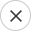• ---------
• 阿富汗
• 阿尔巴尼亚
• 澳大利亚
• 巴西
• 加拿大
• 中国
• 法国
• 德国
• 意大利
• 西班牙
• 瑞士
• 英国
• 美国
• ---------
• 阿富汗
• 阿尔巴尼亚
• 阿尔及利亚
• 美属萨摩亚
• 安道​​尔
• 安哥拉
• 安圭拉
• 安提瓜和巴布达
• 阿根廷
• 亚美尼亚
• 阿鲁巴岛
• 澳大利亚
• 奥地利
• 阿塞拜疆
• 巴哈马
• 巴林
• 孟加拉国
• 巴巴多斯
• 白俄罗斯
• 比利时
• 伯利兹
• 贝宁
• 百慕大
• 不丹
• 玻利维亚
• 波斯尼亚和黑塞哥维那
• 博茨瓦纳
• 布维岛
• 巴西
• 英属印度洋领地
• 文莱达鲁萨兰国
• 保加利亚
• 布基纳法索
• 布隆迪
• 柬埔寨
• 喀麦隆
• 加拿大
• 佛得角
• 开曼群岛
• 中非共和国
• 乍得
• 智利
• 中国
• 圣诞岛
• 科科斯群岛
• 哥伦比亚
• 科摩罗
• 刚果
• 库克群岛
• 哥斯达黎加
• 科特迪瓦
• 古巴
• 塞浦路斯
• 捷克共和国
• 朝鲜人民民主共和国
• 刚果民主共和国
• 丹麦
• 吉布提
• 多明尼加
• 东帝汶
• 厄瓜多尔
• 埃及
• 萨尔瓦多
• 赤道几内亚
• 厄立特里亚
• 爱沙尼亚
• 埃塞俄比亚
• 福克兰群岛
• 法罗群岛
• 斐济
• 芬兰
• 法国
• 法属圭亚那
• 法属波利尼西亚
• 法国南部领土
• 加蓬
• 冈比亚
• 格鲁吉亚
• 德国
• 加纳
• 直布罗陀
• 希腊
• 格陵兰
• 格林纳达
• 瓜德罗普岛
• 关岛
• 危地马拉
• 几内亚
• 几内亚比绍
• 圭亚那
• 海地
• 赫德岛和麦克唐纳群岛
• 洪都拉斯
• 中国香港
• 匈牙利
• 冰岛
• 印度
• 印度尼西亚
• 伊朗
• 伊拉克
• 爱尔兰
• 以色列
• 意大利
• 牙买加
• 日本
• 约旦
• 哈萨克斯坦
• 肯尼亚
• 基里巴斯
• 韩国
• 科威特
• 吉尔吉斯共和国
• 老挝
• 拉脱维亚
• 黎巴嫩
• 莱索托
• 利比里亚
• 列支敦士登
• 立陶宛
• 卢森堡
• 中国澳门
• 马其顿
• 马达加斯加
• 马拉维
• 马来西亚
• 马尔代夫
• 马里
• 马耳他
• 马绍尔群岛
• 马提尼克
• 毛里塔尼亚
• 毛里求斯
• 马约特
• 墨西哥
• 密克罗尼西亚
• 摩尔多瓦
• 摩纳哥
• 蒙古
• 蒙特塞拉特
• 摩洛哥
• 莫桑比克
• 缅甸
• 纳米比亚
• 瑙鲁
• 尼泊尔
• 荷兰
• 新喀里多尼亚
• 新西兰
• 尼加拉瓜
• 尼日尔
• 尼日利亚
• 纽埃
• 诺福克岛
• 北马里亚纳群岛
• 挪威
• 阿曼
• 巴基斯坦
• 帕劳
• 巴勒斯坦
• 巴拿马
• 巴布亚新几内亚
• 巴拉圭
• 秘鲁
• 菲律宾
• 皮特凯恩群岛
• 波兰
• 葡萄牙
• 波多黎各
• 卡塔尔
• 留尼汪岛
• 罗马尼亚
• 俄国
• 卢旺达
• 圣赫勒拿
• 圣基茨和尼维斯
• 圣卢西亚
• 圣皮埃尔和密克隆
• 圣文森特和格林纳丁斯
• 圣马力诺
• 圣多美和普林西比
• 沙特阿拉伯
• 塞内加尔
• 塞尔维亚
• 塞舌尔
• 塞拉利昂
• 新加坡
• 斯洛伐克
• 斯洛文尼亚
• 越南社会主义共和国
• 所罗门群岛
• 索马里
• 南非
• 南乔治亚岛和南桑威奇群岛
• 西班牙
• 斯里兰卡
• 利比亚
• 苏丹
• 苏里南
• 斯瓦尔巴群岛和扬马延岛
• 斯威士兰
• 瑞典
• 瑞士
• 阿拉伯叙利亚共和国
• 中国台湾
• 塔吉克斯坦
• 坦桑尼亚
• 泰国
• 克罗地亚共和国
• 多哥共和国
• 托克劳
• 汤加
• 特立尼达和多巴哥
• 突尼斯
• 土耳其
• 土库曼斯坦
• 特克斯和凯科斯群岛
• 图瓦卢
• 乌干达
• 乌克兰
• 阿拉伯联合酋长国
• 英国
• 美国
• 美国本土外小岛屿
• 乌拉圭
• 美属维尔京群岛
• 乌兹别克斯坦
• 瓦努阿图
• 梵蒂冈城国
• 委内瑞拉
• 瓦利斯和富图纳群岛
• 西撒哈拉
• 萨摩亚
• 也门
• 赞比亚
• 津巴布韦Whatsapp

+86 18923107618